Get inspired by the success stories of our students in IIT JAM MS, ISI  MStat, CMI MSc DS.  Learn More

# ISI MStat PSB 2018 Problem 9 | Regression Analysis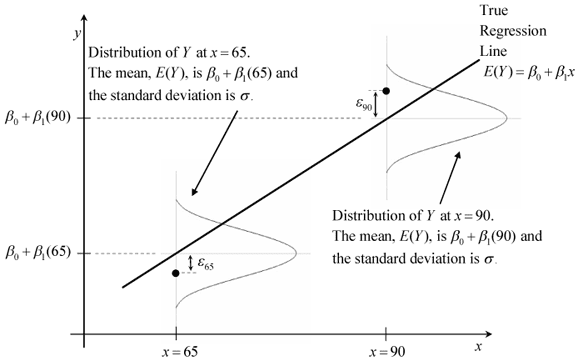This is a very simple sample problem from ISI MStat PSB 2018 Problem 9. It is mainly based on estimation of ordinary least square estimates and Likelihood estimates of regression parameters. Try it!

## Problem - ISI MStat PSB 2018 Problem 9

Suppose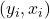satisfies the regression model,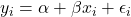for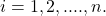where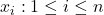are fixed constants and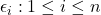are i.i.d.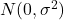errors, where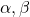andare unknown parameters.

(a) Let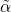denote the least squares estimate of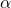obtained assuming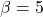. Find the mean squared error (MSE) ofin terms of model parameters.

(b) Obtain the maximum likelihood estimator of this MSE.

### Prerequisites

Normal Distribution

Ordinary Least Square Estimates

Maximum Likelihood Estimates

## Solution :

These problem is simple enough,

for the given model,for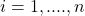.

The scenario is even simpler here since, it is given that, so our model reduces to,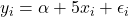, where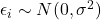and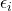's are i.i.d.

now we know that the Ordinary Least Square (OLS) estimate ofis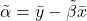(How ??) where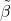is the (generally) the OLS estimate of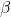, but hereis known, so,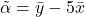again,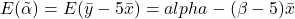, henceis a biased estimator forwith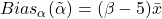.

So, the Mean Squared Error, MSE ofis,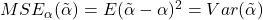+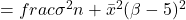[ as, it follows clearly from the model,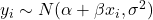and's are non-stochastic ] .

(b) the last part follows directly from the, the note I provided at the end of part (a),

that is,and we have to find the Maximum Likelihood Estimator ofandand then use the inavriant property of MLE. ( in the MSE obtained in (a)). In leave it as an Exercise !! Finish it Yourself !

## Food For Thought

Suppose you don't know the value ofeven, What will be the MSE ofin that case ?

Also, find the OLS estimate ofand you already have done it for, so now find the MLEs of alland. Are the OLS estimates are identical to the MLEs you obtained ? Which assumption induces this coincidence ?? What do you think !!

## Subscribe to Cheenta at Youtube

This is a very simple sample problem from ISI MStat PSB 2018 Problem 9. It is mainly based on estimation of ordinary least square estimates and Likelihood estimates of regression parameters. Try it!

## Problem - ISI MStat PSB 2018 Problem 9

Supposesatisfies the regression model,forwhereare fixed constants andare i.i.d.errors, whereandare unknown parameters.

(a) Letdenote the least squares estimate ofobtained assuming. Find the mean squared error (MSE) ofin terms of model parameters.

(b) Obtain the maximum likelihood estimator of this MSE.

### Prerequisites

Normal Distribution

Ordinary Least Square Estimates

Maximum Likelihood Estimates

## Solution :

These problem is simple enough,

for the given model,for.

The scenario is even simpler here since, it is given that, so our model reduces to,, whereand's are i.i.d.

now we know that the Ordinary Least Square (OLS) estimate ofis(How ??) whereis the (generally) the OLS estimate of, but hereis known, so,again,, henceis a biased estimator forwith.

So, the Mean Squared Error, MSE ofis,+[ as, it follows clearly from the model,and's are non-stochastic ] .

(b) the last part follows directly from the, the note I provided at the end of part (a),

that is,and we have to find the Maximum Likelihood Estimator ofandand then use the inavriant property of MLE. ( in the MSE obtained in (a)). In leave it as an Exercise !! Finish it Yourself !

## Food For Thought

Suppose you don't know the value ofeven, What will be the MSE ofin that case ?

Also, find the OLS estimate ofand you already have done it for, so now find the MLEs of alland. Are the OLS estimates are identical to the MLEs you obtained ? Which assumption induces this coincidence ?? What do you think !!

## Subscribe to Cheenta at Youtube

This site uses Akismet to reduce spam. Learn how your comment data is processed.

### Knowledge Partner